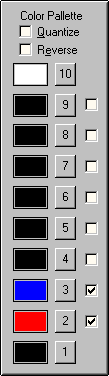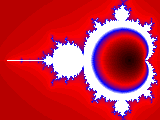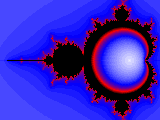PHOTOSWIZZLE Fractals Help - Color Palette## Color Palette` `

To fully understand how the Color Palette works, first read about the Color Scheme and then come back here. After that you will see that basically each pixel in the image has a number assigned to it after the fractal equation calculations are done.

When the calculations have been completed on all pixels, Fractals figures out which one took the most iterations to complete. Color 10 is assigned to the maximum number. All other pixel colors are then assigned linearly based on the ratio of how many iterations they took to complete relative to the maximum number.

The color assignments also depend on how many colors in the color palette are enabled. Colors 1 and 10 are always enabled so there are always at least 2. For example, if only colors 2 and 3 are enabled, then 4 colors total are used to create the image. If the maximum number of iterations for any pixel is 60, then any pixel that took 60 iterations to calculate is color 10. For all pixels that took 40 iterations to complete the color is 3, 20 iterations is color 2 and 1 iteration (minimum) is color 1.

Here's where the Quantize control comes into play. If Quantize is not selected then all other pixels colors are assigned on a continuum between the dividing points. To continue with the same example above, for pixels that took 30 iterations to complete the pixel color is a blend half way between colors 2 and 3. If Quantize is selected, all pixel colors are assigned to the exact color in the palette which corresponds to the nearest lower dividing point. So all pixels that took between 20 and 39 iterations to complete are assigned color 2.

The last control in this group is Reverse. If Reverse is selected the colors are assigned in the reverse order, i.e effectively the palette is flipped upside down. For example, here's the default settings with Quantize or Reverse selected:Quantize selectedDefault settingsReverse selected

```
```